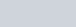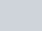If the probability of winning a game is 0.3,
Question:

If the probability of winning a game is 0.3,  what is the probability of loosing it?

Solution:

Given: Probability of winning a gameTO FIND: Probability of losing the gameCALCULATION: We know that sum of probability of occurrence of an event and probability of non occurrence of an event is 1.

$P(E)+P(\bar{E})=1$

$0.3+P(\bar{E})=1$

$P(\bar{E})=1-0.3$

$P(\bar{E})=0.7$

Hence the probability of losing the game is $P(\bar{E})=0.7$